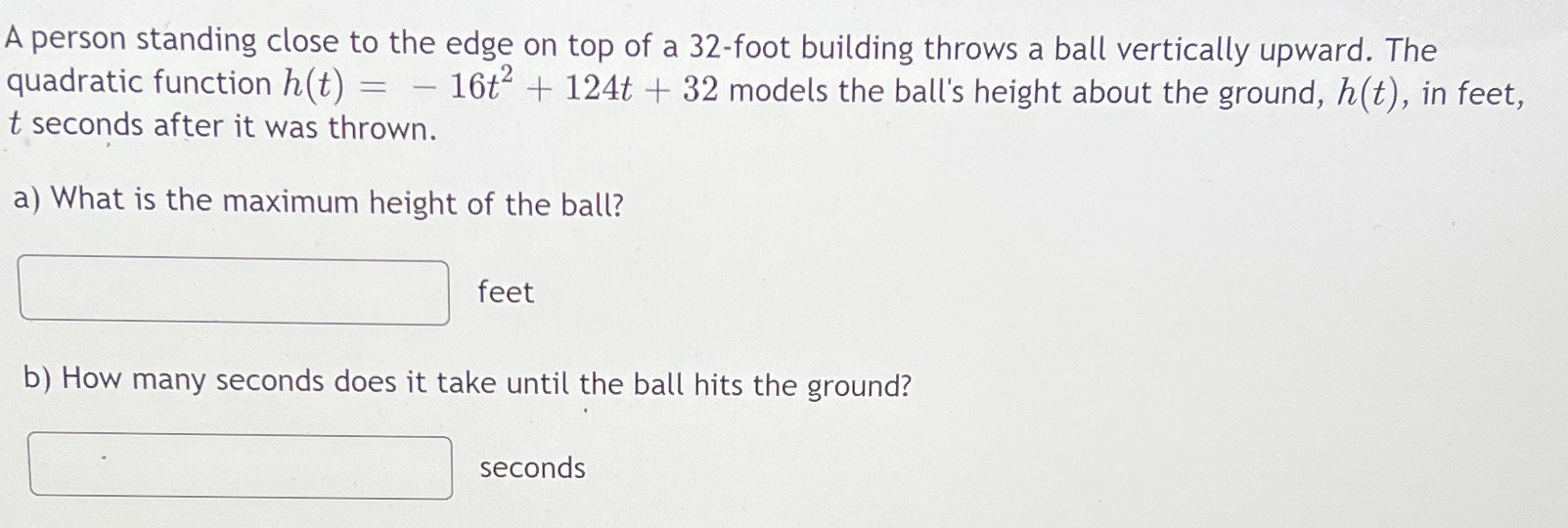### ¿Todavía tienes preguntas de matemáticas?

Pregunte a nuestros tutores expertos
Algebra
PreguntaA person standing close to the edge on top of a $$32$$ -foot building throws a ball vertically upward. The quadratic function $$h ( t ) = - 16 t ^ { 2 } + 124 t + 32$$ models the ball's height about the ground, $$h ( t )$$ , in feet, $$t$$ seconds after it was thrown.

a) What is the maximum height of the ball? $$\square$$ feet

b) How many seconds does it take until the ball hits the ground? $$\square$$ seconds